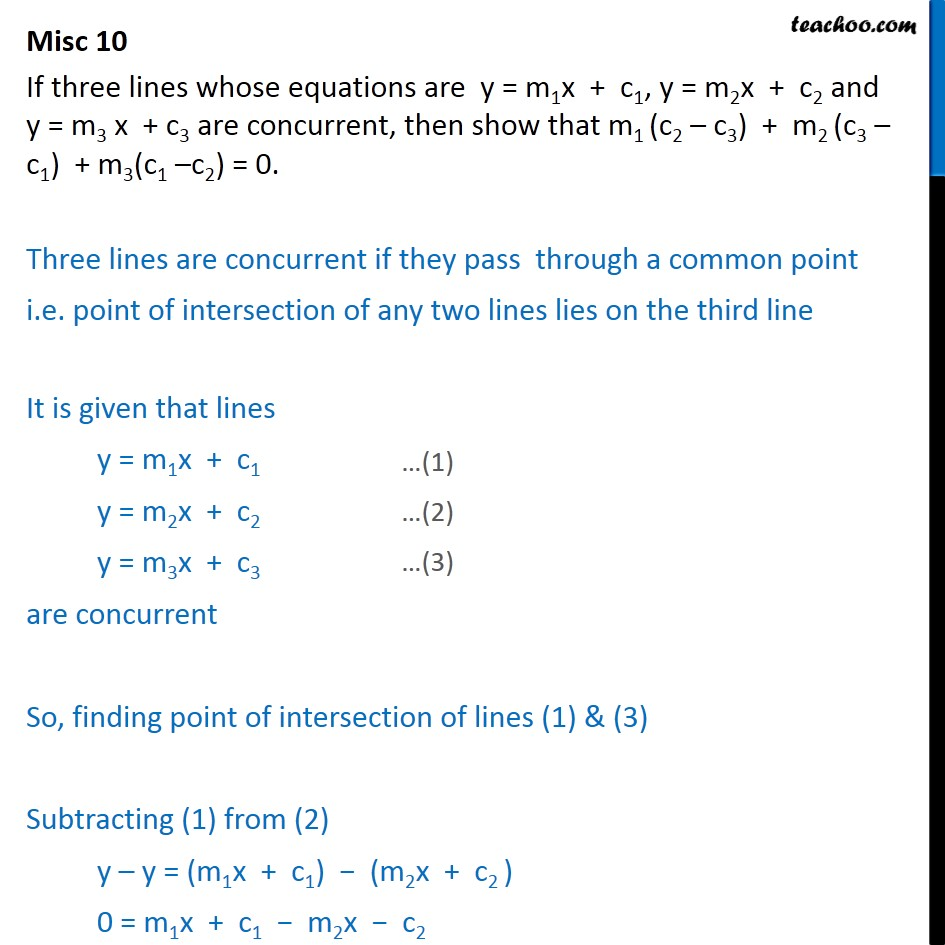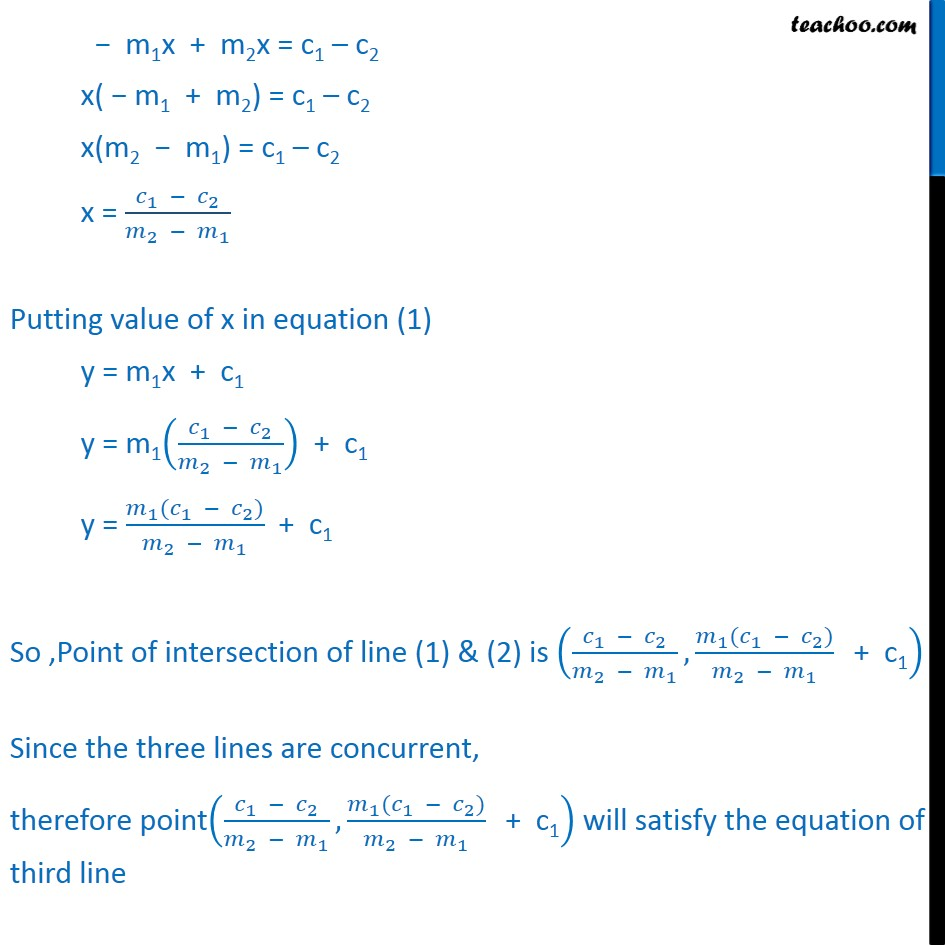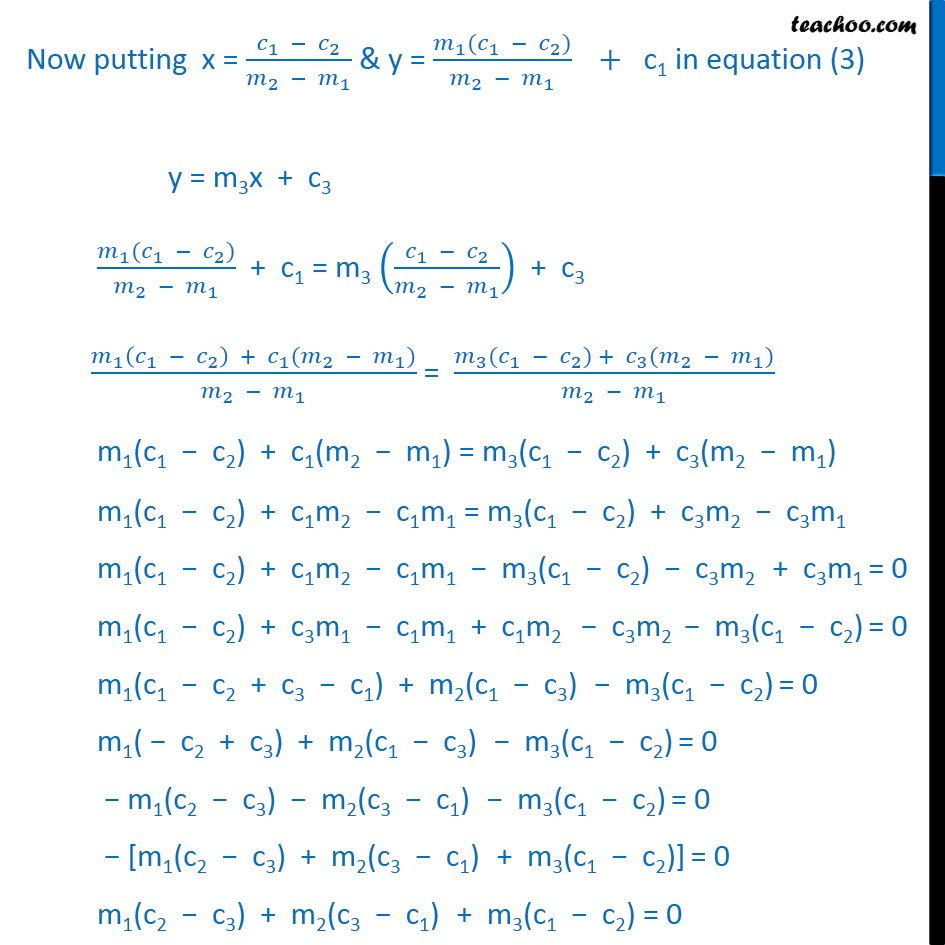Miscellaneous

Chapter 9 Class 11 Straight Lines
Serial order wiseLearn in your speed, with individual attention - Teachoo Maths 1-on-1 Class

### Transcript

Misc 9 If three lines whose equations are y = m1x + c1, y = m2x + c2 and y = m3 x + c3 are concurrent, then show that m1 (c2 – c3) + m2 (c3 – c1) + m3(c1 –c2) = 0. Three lines are concurrent if they pass through a common point i.e. point of intersection of any two lines lies on the third line It is given that lines y = m1x + c1 y = m2x + c2 y = m3x + c3 are concurrent So, finding point of intersection of lines (1) & (3) Subtracting (1) from (2) y – y = (m1x + c1) − (m2x + c2 ) 0 = m1x + c1 − m2x − c2 − m1x + m2x = c1 – c2 x( − m1 + m2) = c1 – c2 x(m2 − m1) = c1 – c2 x = (𝑐_1 − 〖 𝑐〗_2)/(𝑚_2 − 𝑚_1 ) Putting value of x in equation (1) y = m1x + c1 y = m1((𝑐_1 − 〖 𝑐〗_2)/(𝑚_2 − 𝑚_1 )) + c1 y = (𝑚_1 (𝑐_1 − 𝑐_2))/(𝑚_2 〖 − 𝑚〗_1 ) + c1 So ,Point of intersection of line (1) & (2) is ((𝑐_1 − 〖 𝑐〗_2)/(𝑚_2 − 𝑚_1 ),(𝑚_1 (𝑐_1 − 𝑐_2))/(𝑚_2 〖 − 𝑚〗_1 ) " + c1" ) Since the three lines are concurrent, therefore point((𝑐_1 − 〖 𝑐〗_2)/(𝑚_2 − 𝑚_1 ),(𝑚_1 (𝑐_1 − 𝑐_2))/(𝑚_2 〖 − 𝑚〗_1 ) " + c1" ) will satisfy the equation of third line Now putting x = (𝑐_1 − 〖 𝑐〗_2)/(𝑚_2 − 𝑚_1 ) & y = (𝑚_1 (𝑐_1 − 𝑐_2))/(𝑚_2 〖 − 𝑚〗_1 ) " " + " c1" in equation (3) y = m3x + c3 (𝑚_1 (𝑐_1 − 𝑐_2))/(𝑚_2 〖 − 𝑚〗_1 ) + c1 = m3 ((𝑐_1 − 〖 𝑐〗_2)/(𝑚_2 − 𝑚_1 )) + c3 (𝑚_1 (𝑐_1 − 𝑐_2 ) + 〖 𝑐〗_1 (𝑚_2 − 𝑚_1))/(𝑚_2 〖 − 𝑚〗_1 ) = (〖𝑚_3 (𝑐〗_1 − 〖 𝑐〗_2) + 〖 𝑐〗_3 (𝑚_2 − 𝑚_1))/(𝑚_2 − 𝑚_1 ) m1(c1 − c2) + c1(m2 − m1) = m3(c1 − c2) + c3(m2 − m1) m1(c1 − c2) + c1m2 − c1m1 = m3(c1 − c2) + c3m2 − c3m1 m1(c1 − c2) + c1m2 − c1m1 − m3(c1 − c2) − c3m2 + c3m1 = 0 m1(c1 − c2) + c3m1 − c1m1 + c1m2 − c3m2 − m3(c1 − c2) = 0 m1(c1 − c2 + c3 − c1) + m2(c1 − c3) − m3(c1 − c2) = 0 m1( − c2 + c3) + m2(c1 − c3) − m3(c1 − c2) = 0 − m1(c2 − c3) − m2(c3 − c1) − m3(c1 − c2) = 0 − [m1(c2 − c3) + m2(c3 − c1) + m3(c1 − c2)] = 0 m1(c2 − c3) + m2(c3 − c1) + m3(c1 − c2) = 0 Hence proved Hence proved